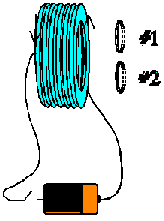Teaching Physics with the Physics Suite Edward F. Redish

Inducing current

 In the picture at the right is shown a solenoid and two loops. When the switch is closed, the solenoid carries a current in the direction indicated. The planes of the loops are parallel to the planes of the loops of the solenoid. The picture is drawn so that the part of the loop closest to you is drawn as solid, while that part farthest away is drawn dashed. Loop #1 consists of a single turn of resistive wire that has a resistance per unit length of l. Loop #2 consists of NĀturns of the same wire. Each loop is a circle of radius r The switch is closed and remains closed for a few seconds. Loop #1 is then moved to the right. Is there a current flow induced? If there is a current, indicate the direction and explain how you figured it out.1. The loops are now returned to their original locations and held fixed. The switch is opened. For a short time, the magnetic field at the loops decreases like

B = B0 - gt

where g is a constant. Is there a current flow induced in the loops? If there is a current, indicate the direction

2. Calculate the current flow in each loop for situation (b).
3. If B0 = 10 G, g  = 2.5 G/s, the resistivity of the wire is 1 microOhm/m, and the loops have a radius of 2 cm, calculate the current induced in loop #1 as the B field from the solenoid begins to fall.

Note to the instructor: This problem combines qualitative material from a UW Tutorial [Tutorials 1998] with quantitative calculations. Something like this is needed to help students bridge qualitative and quantitative reasoning. Too often, they tend to think of these as separate and distinct approaches to problem solving.

Not finding what you wanted? Check the Site Map for more information.